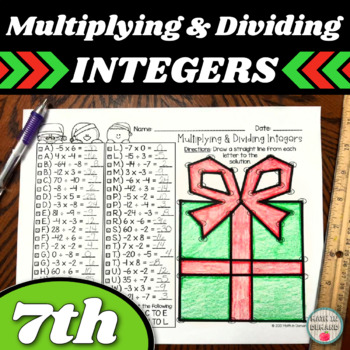# Multiplying and Dividing Integers Letter to Number Christmas 7th Grade Math;
6th - 8th
Subjects
Resource Type
Formats Included
• PDF
Pages
2 pages
Report this resource to TPT
##### Also included in
1. There are 2 worksheets: (1) Adding &amp; Subtracting Integers and (2) Multiplying &amp; Dividing Integers.#1 - Adding &amp; Subtracting IntegersStudents will solve 55 problems on adding and subtracting integers. After solving the problems, students will draw a line from the letter to the solution. O
Price \$4.50Original Price \$5.00Save \$0.50
2. This is a bundle on integer operations. There are currently 8 resources on adding &amp; subtracting integers and multiplying &amp; dividing integers. This includes:1.) Adding &amp; Subtracting Integers Halloween Frankenstein Mystery Picture2.) Multiplying &amp; Dividing Integers Halloween Jack O' La
Price \$18.90Original Price \$21.00Save \$2.10

### Description

Students will solve 41 problems on multiplying & dividing integers. After solving the problems, students will draw a line from the letter to the solution. Once all the lines are drawn a mystery picture will be revealed. This picture is a Christmas present. This resource is perfect for Christmas!

Perfect for 6th Grade Math, 7th Grade Math, or 8th Grade Math classrooms learning how to multiply and divide integers.

Click the green "PREVIEW" button to see the resource close up!

Total Pages
2 pages